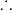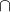##### Question

In: Statistics and Probability

# Two fair coins and a fair die are tossed. Find the sample space of the experiment...

Two fair coins and a fair die are tossed. Find the sample space of the
experiment (10 pts); Find the probabilities of the following events:
A- ”the die shows 2 or 3” (5 pts);
B- ”one of the coins is head, the other - tail, and the die shows an odd number” (5
pts).
Are the events A and B independent? (5 pts).
Give proofs.

## Solutions

##### Expert Solution

solution:

When two fair coins tossed,we have

sample space s1 = { HH,HT,TH,TT }

when a die is thrown, we have

sample space s2 = {1,2,3,4,5,6}

In the event Two fair coins and a fair die is Tossed.we have the following samle space

Sample space S = { HH1,HH2,HH3,HH4,HH5,HH6,

HT1,HT2,HT3,HT4,HT5,HT6,

TH1,TH2,TH3,TH4,TH5,TH6,

TT1,TT2,TT3,TT4,TT5,TT6 }

n(S) = 24

a) Let A = event that die shows 2 or 3 = {HH2,HH3,HT2,HT3,TH2,TH3,TT2,TT3 }

n(A) = 8

P(  die shows 2 or 3 ) = P(A)

= n(A) / n(S)

= 8 / 24

= 0.33Probability that  die shows 2 or 3 = 0.3333

b) Let B = one of the coins is head, the other - tail, and the die shows an odd number

= { HT1,HT3,HT5,TH1,TH3,TH5 }

n(B) = 6P (one of the coins is head, the other - tail, and the die shows an odd number )= P(B)

= n(B) / n(S)

= 6 / 24

= 0.25

Probability that  one of the coins is head, the other - tail, and the die shows an odd number = 0.25

c) If two events A and B are independent then P(A|B) = P(A)

i.e., P(AB) = P(A) * P(B)

Here, AB = {HH2,HH3,HT2,HT3,TH2,TH3,TT2,TT3 }{ HT1,HT3,HT5,TH1,TH3,TH5 }

= { HT3,TH3 }

n( AB) = 2

P(AB) = n( AB) / n(S) = 2/24 = 1/12 =0.0833

P(A) * P(B) = 0.3333 * 0.25

= 0.0833

=  P(AB)P(AB) = P(A) * P(B)A and B are independent events

## Related Solutions

##### Two coins are tossed and a (6-sided) die is rolled. Describe a sample space(probability space), together...
Two coins are tossed and a (6-sided) die is rolled. Describe a sample space(probability space), together with the probability, on which such a situation can be modeled. Find the probability mass function of the random variable whose value is the sum of the number on the die and the total number of heads.
##### Consider an experiment where fair die is rolled and two fair coins are flipped. Define random...
Consider an experiment where fair die is rolled and two fair coins are flipped. Define random variable X as the number shown on the die, minus the number of heads shown by the coins. Assume that all dice and coins are independent. (a) Determine f(x), the probability mass function of X (b) Determine F(x), the cumulative distribution function of X (write it as a function and draw its plot) (c) Compute E[X] and V[X]
##### Two fair dice are tossed, and the up face on each die is recorded. Find the...
Two fair dice are tossed, and the up face on each die is recorded. Find the probability of observing each of the following events: A: { The sum of the numbers is even } B: { A 4 appears on at least one of the dice } C: { The difference of the numbers is 2 or less }
##### Two coins are tossed. A die is thrown for each coin that falls heads. What is...
Two coins are tossed. A die is thrown for each coin that falls heads. What is the expected number of spots shown on the dice?
##### 1. (a) List the sample space in the experiment "roll two dice - a red die...
1. (a) List the sample space in the experiment "roll two dice - a red die and a white die." (Hint: Use a table) (b) Find the probability that the sum of the numbers on the two dice is less than seven. (c) Find the probability that the sum of the numbers on the two dice is odd.
##### (A) Suppose two coin are tossed, determine the sample space and find two sigma field over...
(A) Suppose two coin are tossed, determine the sample space and find two sigma field over the sample. (B) From the experiment also give example of a set that is not a sigma field (C) With examples, define and explain in details the concept of (a) Probability space (b) Probability Measure (c) sigma algebra or sigma field
##### A fair coin is tossed, and a fair die is rolled. Let H be the event...
A fair coin is tossed, and a fair die is rolled. Let H be the event that the coin lands on heads, and let S be the event that the die lands on six. Find P(H or S).
##### Two fair dice are tossed, and the face on each die is observed. Use a tree...
Two fair dice are tossed, and the face on each die is observed. Use a tree diagram to find the 36 sample points contained in the sample space. Assign probabilities to the sample points in part a. Find the probability of each of the following events: A = {3 showing on each die} B = {sum of two numbers showing is 7} C = {sum of two numbers showing is even} Also, for events A, B, and C, which one...
##### A fair coin is tossed 4 times. a. Write the outcomes of the sample space b....
A fair coin is tossed 4 times. a. Write the outcomes of the sample space b. Let A be the event of obtaining at least one head, find P(A) c. Let B be the event of obtaining at least one tail, find P(B) d. Let C be the event of obtaining two tails, find P(C) e. Find P(A ∪ B) f. Find P(A ∩ B) g. Find P(A ∪ B ∪ C)
##### Three fair coins are tossed simultaneously 10 times. Find the probability that "2 heads and one...
Three fair coins are tossed simultaneously 10 times. Find the probability that "2 heads and one tail" will show up (a) at least once and (b) at most once.